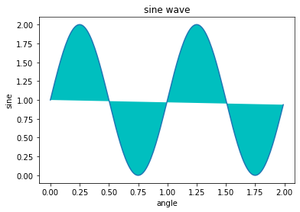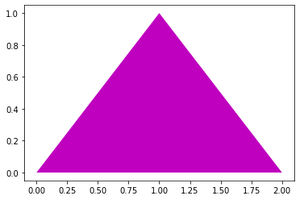# Matplotlib.pyplot.fill() function in Python

• Last Updated : 25 Nov, 2020

Matplotlib.pyplot.fill() function is used to fill the area enclosed by polygon /curve.

Syntax: matplotlib.pyplot.fill(*args, data=None, **kwargs)

Parameters:
*args: sequence of x, y, [color]
sequence of x,y = To traverse the boundaries of the polygon or curve defined by lists of x and y positions of its nodes.color = To change the default fill color to the desired one.You can plot multiple polygons by providing multiple x, y, [color] groups.
data: indexable object, optional
default value = none
You can directly provide labeled data in the form of a dictionary. For better understanding refer to Example2

Returns: A list of Polygon

Other Parameters:
**kwargs: Supports all other properties of Polygon patch.

Example 1:

## Python3

 `# Importing the library``import` `matplotlib``import` `matplotlib.pyplot as plt``import` `numpy as np`` ` `# Data for plotting``x ``=` `np.arange(``0.0``, ``2.0``, ``0.01``)``y ``=` `1` `+` `np.sin(``2` `*` `np.pi ``*` `x)``plt.plot(x, y)`` ` `# Assighning plot attributes``plt.xlabel(``"angle"``)``plt.ylabel(``"sine"``)``plt.title(``'sine wave'``)`` ` `# Filling sign wave curv with cyan color``plt.fill(x, y, ``"c"``)``plt.show()`

Output:Example 1_Output_GFG

Example 2:

## Python3

 `# Importing libraries``import` `matplotlib``import` `matplotlib.pyplot as plt`` ` ` ` `# Below we are using data attribute``plt.fill(``"j"``, ``"k"``, ``'m'``,``         ``data``=``{``"j"``: [``0``, ``1``, ``2``],``               ``"k"``: [``0``, ``1``, ``0``]})  ``# here 'm' for magenta`

Output:Example 2_Output_GFG

My Personal Notes arrow_drop_up